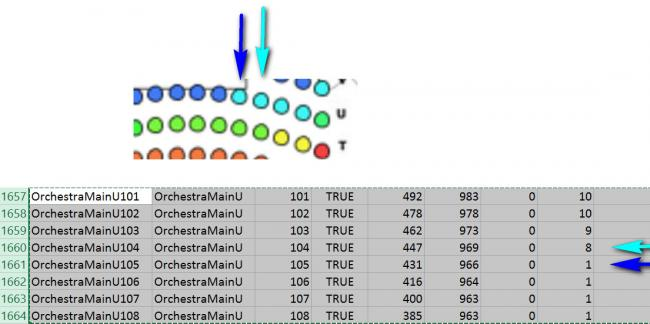#### Howdy, Stranger!

We are about to switch to a new forum software. Until then we have removed the registration on this forum.

# Why does the displayed image seem to be using the i - 1 row rather than i?

edited August 2017

The idea is to display 1677 ellipses with certain colors dependent upon the 'heat/heet' value generated by using the data tables imported from some csv files. I have created output files to confirm the table data is being calculated as expected, however the generated image seems to be using the color of the PRIOR row (i minus 1) in the table, rather than the same row. I've excerpted the generated image and heat.csv table to illustrate.

I'm thinking the structure of the program is somehow causing this. I have tested my for loops and all the indexes seem to be correct at first glance.

``````import java.util.Arrays;
import processing.pdf.*;
PImage img;
Seat[] seats;
Table locsTable;
Table tktdTable;

void setup() {
size(786, 1524);
}

void draw() {
image(img, 0, 0);
// Display all seats
for (int i = 0; i<seats.length; i++) {
seats[i].display();
/// PROBLEM: When displaying ellipses the value 'heet' seems to be using the 'heatcount' value from the i - 1 row instead of the i row.
}
}

int countOccurrence(String seatArea, Table tableOfSeats) {
int count = 0;
for (int i = 0; i <tableOfSeats.getRowCount(); i++) {
if (seatArea.equalsIgnoreCase(tableOfSeats.getString(i, 0))) {
count++;
}
}
return count;
}

// "header" indicates the file has header row. The size of the array
// is then determined by the number of rows in the table.

seats = new Seat[locsTable.getRowCount()];

for (int i = 0; i<tktdTable.getRowCount(); i++) {
// Iterate over all the rows in the Ticketed table.
int heatcount = countOccurrence(tktdTable.getString(i, 0), tktdTable);
tktdTable.setInt(i, "timestktd", heatcount);
}

for (int i = 0; i < tktdTable.getRowCount(); i++) {
TableRow rowt = tktdTable.getRow(i);
//Test to see what the first indexed row is
// TableRow rowone = tktdTable.getRow(0);
//String sone = rowone.getString("seatarea");
//println(sone);
int match = locsTable.findRowIndex(rowt.getString("seatarea"), "seatarea");
// if (match < 0) {
//   println(i, match);
//  match = 0;
// }

locsTable.setInt(match, "heatcount", rowt.getInt("timestktd"));
}

for (int i = 0; i<locsTable.getRowCount(); i++) {
// Iterate over all the rows in the Locations table.
TableRow row = locsTable.getRow(i);
//Test to see what the x indexed row is
//TableRow rowone = locsTable.getRow(1676);
//String sone = rowone.getString("seatarea");
//String sheat = rowone.getString("heatcount");
//println(sone + " " + sheat);

// Access the fields via their column name (or index).
float x = row.getFloat("coordX");
float y = row.getFloat("coordY");
int heat = row.getInt("heatcount");
//println(i,x,y,heat);
// Make a Seat object out of the data from each row.
seats[i] = new Seat(x, y, heat);
}

saveTable(tktdTable, "data/counted.csv");
saveTable(locsTable, "data/heat.csv");
}

class Seat {
float u, v;
int heet;

Seat(float tempu, float tempv, int tempheet) {
u = tempu;
v = tempv;
heet = tempheet;
}

void display() {
// PROBLEM: When displaying ellipses the value 'heet' seems to be using the 'heatcount' value from the i - 1 row instead of the i row.
ellipse(u, v, 11, 11);

if (heet >= 5 && heet <= 12) {
fill(66, 244, 244);
} else if (heet >= 13 && heet <= 20) {
fill(66, 244, 122);
} else if (heet >= 21 && heet <= 28) {
fill(66, 244, 66);
} else if (heet >= 29 && heet <= 36) {
fill(122, 244, 66);
} else if (heet >= 37 && heet <= 44) {
fill(244, 244, 66);
} else if (heet >= 45 && heet <= 52) {
fill(244, 122, 66);
} else if (heet >= 53) {
fill(244, 66, 66);
} else {
fill(66, 122, 244);
}
}
}
``````Tagged: